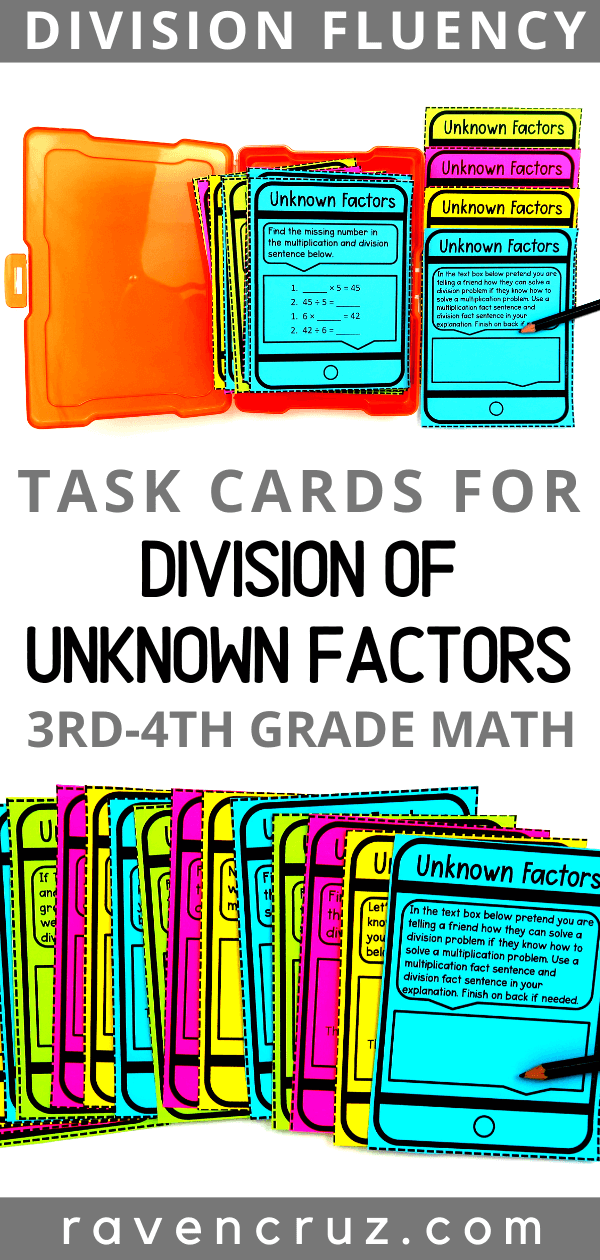Division as an Unknown Factor for 3rd Grade Math - Raven Cruz

# Division as an Unknown Factor for 3rd Grade Math

For students to understand division as an unknown factor, they must first understand the factors and product that correspond with the factors and quotient. For example,

for a student to be able to solve:

45 ÷ 9

they should first understand that:

9 × 5 = 45

There are two ways that I like to teach division as an unknown factor; arrays and fact families.## Using Arrays and Division as an Unknown Factor

Arrays are a multiplication and division strategy that help students visualize and develop a stronger sense of multiplication and division. Also, arrays are useful to help students represent their thinking with manipulatives like counters, cubes, etc...